# Basics of Antenna Array For Beginners

## Basics of Antenna Arrays

An antenna array is an assembly of radiating elements. Radiation pattern of a single element is relatively wide, each element provides low values of directivity. However, in many applications we require antennas with very high directive characteristics. The directive characteristics of the antennas can be improved by increasing the electrical size of the antenna.

One way to increase the dimension of the antenna without necessarily increasing the size of the individual elements is to form an array of antenna elements. The total field of the array is determined by the vector addition of the fields radiated by the individual elements. The elements of the array need not be identical, but it is often convenient and simpler to design such arrays when the individual elements are considered to be identical.

Therefore, here we will consider an array with identical elements. In designing arrays we have several controls such as geometrical configuration of the overall array, distance between the elements, excitation (amplitude and phase) and pattern of individual elements.

## Array of identical elements

In this section, we establish the basic methodology for analyzing an array of identical elements.

As shown in figure, let us consider an array of N identical elements. The position vector of the ith element is given by . The excitation of ith element is given  where  and  are respectively the relative amplitudes and phases.

Let the electric field radiated by an element, when placed at the origin and with an unity excitation is given by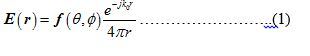The distance from the ith element to the far field point of interest is  for phase variation and  for amplitude variation.The total electric field at the point P is given by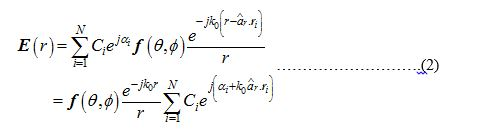As can be seen from (2), the total radiation field is given by the product of the radiation field of the reference element and the term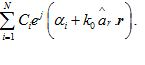The term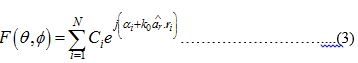is called the array factor of the antenna array.

## Two element array

In equation (3) we observed the expression for the array factor for an N- element array. To simplify our discussion, let us consider a two-element array. Further, we consider the elements are to be isotropic point sources. The array configuration under consideration is shown in Figure below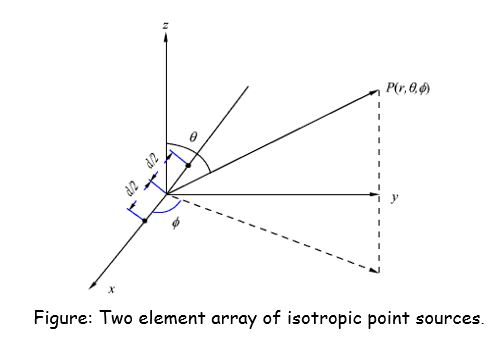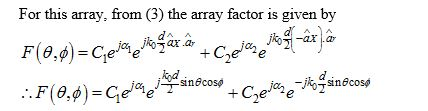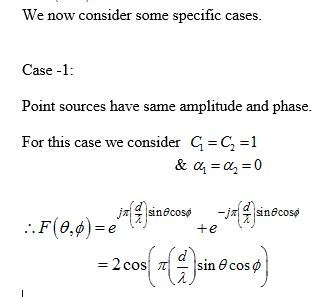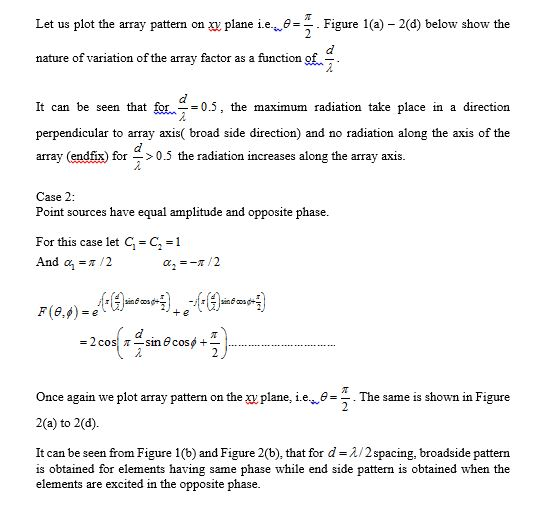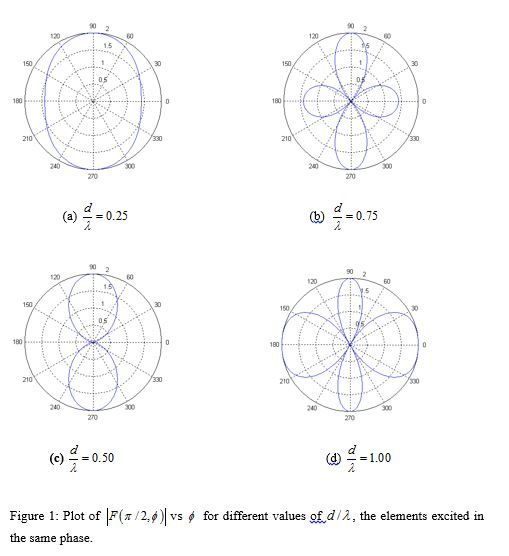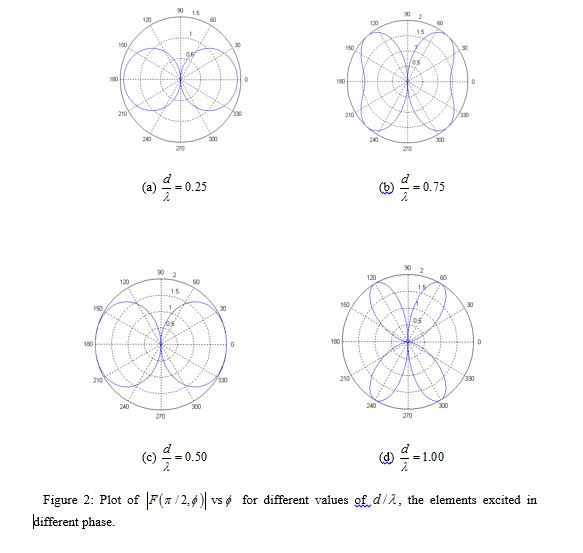Characteristics

The main purpose of creating a fixed antenna array is an increase in the antenna’s directional gain; an array consisting of N identical elements can achieve an increase in gain of up to a factor of N if optimally fed. A parasitic array does not achieve such an increase since there are more constraints on the distribution of currents attainable through passive coupling.

Use of arrays for increasing antenna gain are an alternative to so-called aperture antennas, in which the gain is achieved using a single geometric structure, more akin to an optical focusing device. Designs such as the horn antenna and parabolic dish antenna become more practical at higher frequencies (shorter wavelengths) whereas antenna arrays may be practical at any wavelength. At lower frequencies, where rigidly turning a large structure may be unfeasible, an array may be made steerable (or may just be tuned to optimize a particular radiation pattern) through electronic adjustment of the elements’ relative phases. This principle is often used in AM broadcast, where two or more large fixed towers (vertical monopoles) may be “phased up” to create a desired radiation pattern; obviously such structures cannot be moved once erected.